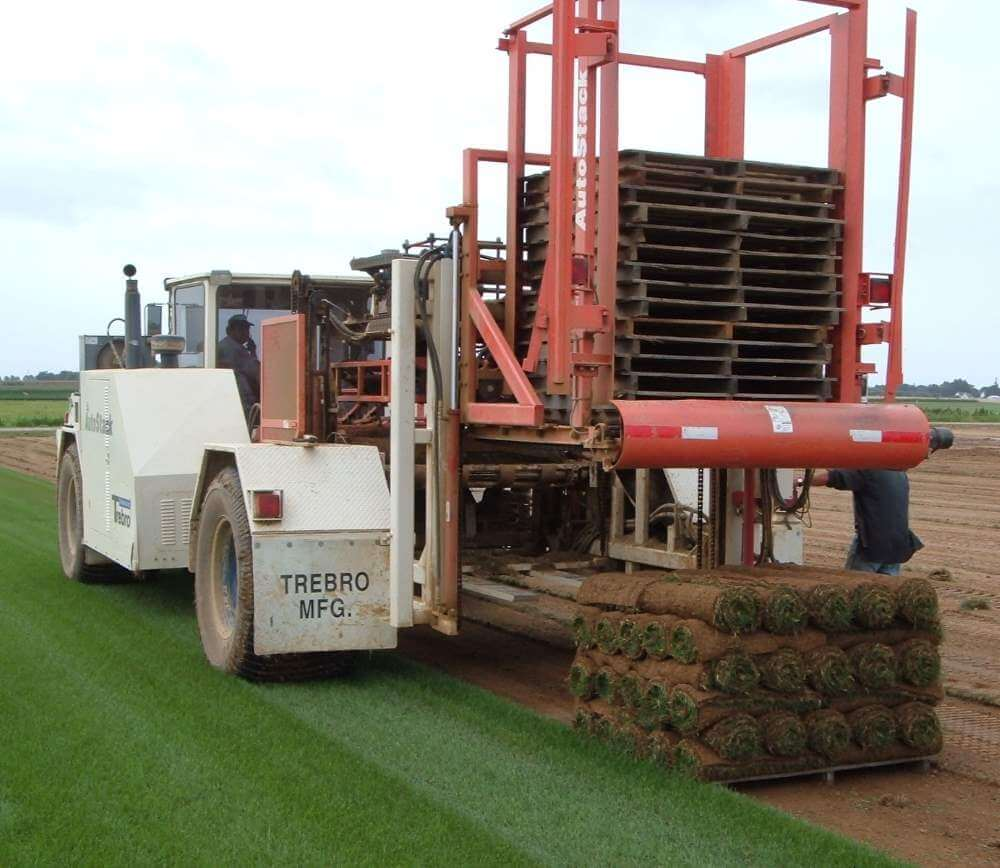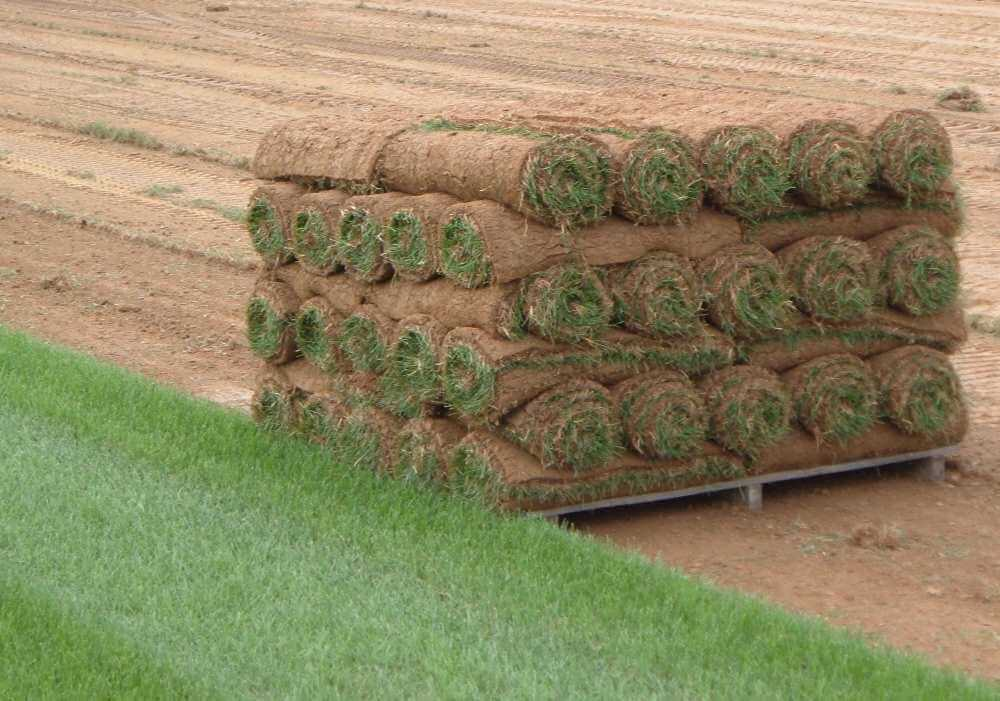# Sod Calculator

Created by Wojciech Sas, PhD
Reviewed by Bogna Szyk and Steven Wooding
Last updated: Jun 05, 2023

This sod calculator is a very helpful tool that tells you how many rolls of sod you need to buy and how much it will cost to cover the given square footage with this particular type of grass. Read below about the sod's structure, find the answer to the question: "How much sod do I need?" and estimate the final cost of a sod lawn.

🙋 Omni's square footage calculator may also be handy while working out the total area of the field.

## What is sod?

Are you seeding grass or laying sod? This is one of the first questions that comes to mind when you try to organize the space reserved for a lawn. During landscape gardening, you will face many similar challenges, e.g., if you want to design the perfect plant spacing. The sod (or turf) is a prevalent type of swards that you can put either around a residential home or some vast open areas like golf courses and sports fields.Sod consists of a specific type of grass and a layer of soil beneath, which provides the root system to be held in a compact form. Depending on the cultivation climate, there can also be a mixture of different grass species. Sod is grown and then harvested as an ordinary crop for sale. After removing grass from the ground, it is usually stored in the form of rolls.

🙋 If you need extra soil beneath the sod, our soil calculator would be helpful!

One of the most significant advantages of sod is that we can quickly spread it out on the ground on a horizontal surface or a slope, and the laying time during the season isn't as crucial as for seeding. On the other hand, it's relatively expensive compared to the alternative, but at the same time, the maintenance is much cheaper, and the problem of weeds is greatly reduced.

## How to estimate the total cost of sod?

"How much sod do I need to fill the terrain?" – we can find the answer in a relatively simple way. We can divide the calculation process into a few steps. Before we start, ensure that you take care of the stable and durable substrate underneath. The reasonable choice could be gravel or a sand base.

Let's assume we want to cover a rectangular field, which is 130 ft long and 50 ft wide.

1. Calculate the area of the land, which we can approximate as an area of a rectangle:

130 ft × 50 ft = 6,500 ft².

2. Take into consideration the waste factor p, which relates to the wasted material in merging pieces. It's usually given as a percentage of the total area. In this sod calculator, we take p = 10% by default, but it's worth considering values up to 20% if the field contains numerous round and protruding elements, e.g., trees or rocks.

3. Estimate the total area of sod:

6,500 ft² × (1 + p) = 7,150 ft².

4. Suppose that we use 1.5 × 6 ft sod pieces, we can work out the number of rolls:

7,150 ft² / (1.5 ft × 6 ft) = 794.4.

5. If we want to buy rolls with the price of 0.25 $/ft², then the total cost of sod can be found: 7,150 ft² × 0.25$/ft² = $1,787.5. Once you want to use square sod elements, the GCF calculator can be handy if you are interested in the biggest possible size of a single element. Sometimes, the field doesn't have a regular shape, but it's not a problem – try our area of a trapezoid calculator and save you time!Wojciech Sas, PhD Field parameters Length m Width m Total area Waste factor % Results Number of rolls Roll price$/
ft²
Total cost
\$
People also viewed…

### Cat age

If you're curious what is the age of your cat in human years, try this cat age calculator.

### Humans vs vampires

Vampire apocalypse calculator shows what would happen if vampires were among us using the predator - prey model.

### Significant figures

The significant figures calculator performs operations on sig figs and shows you a step-by-step solution!

### Tree spacing

This tree spacing calculator tells you how many trees you can plant in your field or garden.